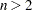The FREQ Procedure
 Output Data Sets

PROC FREQ produces two types of output data sets that you can use with other statistical and reporting procedures. You can request these data sets as follows:

• Specify the OUT= option in a TABLES statement. This creates an output data set that contains frequency or crosstabulation table counts and percentages

• Specify an OUTPUT statement. This creates an output data set that contains statistics.

PROC FREQ does not display the output data sets. Use PROC PRINT, PROC REPORT, or any other SAS reporting tool to display an output data set.

In addition to these two output data sets, you can create a SAS data set from any piece of PROC FREQ output by using the Output Delivery System. See the section ODS Table Names for more information.

### Contents of the TABLES Statement Output Data Set

The OUT= option in the TABLES statement creates an output data set that contains one observation for each combination of variable values (or table cell) in the last table request. By default, each observation contains the frequency and percentage for the table cell. When the input data set contains missing values, the output data set also contains an observation with the frequency of missing values. The output data set includes the following variables:

• BY variables

• table request variables, such as A, B, C, and D in the table request A*B*C*D

• COUNT, which contains the table cell frequency

• PERCENT, which contains the table cell percentage

If you specify the OUTEXPECT option in the TABLES statement for a two-way or multiway table, the output data set also includes expected frequencies. If you specify the OUTPCT option for a two-way or multiway table, the output data set also includes row, column, and table percentages. The additional variables are as follows:

• EXPECTED, which contains the expected frequency

• PCT_TABL, which contains the percentage of two-way table frequency, for n-way tables where• PCT_ROW, which contains the percentage of row frequency

• PCT_COL, which contains the percentage of column frequency

If you specify the OUTCUM option in the TABLES statement for a one-way table, the output data set also includes cumulative frequencies and cumulative percentages. The additional variables are as follows:

• CUM_FREQ, which contains the cumulative frequency

• CUM_PCT, which contains the cumulative percentage

The OUTCUM option has no effect for two-way or multiway tables.

The following PROC FREQ statements create an output data set of frequencies and percentages:

```    proc freq;
tables A A*B / out=D;
run;
```

The output data set D contains frequencies and percentages for the table of A by B, which is the last table request listed in the TABLES statement. If A has two levels (1 and 2), B has three levels (1,2, and 3), and no table cell count is zero or missing, then the output data set D includes six observations, one for each combination of A and B levels. The first observation corresponds to A=1 and B=1; the second observation corresponds to A=1 and B=2; and so on. The data set includes the variables COUNT and PERCENT. The value of COUNT is the number of observations with the given combination of A and B levels. The value of PERCENT is the percentage of the total number of observations with that A and B combination.

When PROC FREQ combines different variable values into the same formatted level, the output data set contains the smallest internal value for the formatted level. For example, suppose a variable X has the values 1.1., 1.4, 1.7, 2.1, and 2.3. When you submit the statement

```    format X 1.;
```

in a PROC FREQ step, the formatted levels listed in the frequency table for X are 1 and 2. If you create an output data set with the frequency counts, the internal values of the levels of X are 1.1 and 1.7. To report the internal values of X when you display the output data set, use a format of 3.1 for X.

### Contents of the OUTPUT Statement Output Data Set

The OUTPUT statement creates a SAS data set that contains the statistics that PROC FREQ computes for the last table request. You specify which statistics to store in the output data set. There is an observation with the specified statistics for each stratum or two-way table. If PROC FREQ computes summary statistics for a stratified table, the output data set also contains a summary observation with those statistics.

The OUTPUT data set can include the following variables.

• BY variables

• variables that identify the stratum, such as A and B in the table request A*B*C*D

• variables that contain the specified statistics

The output data set also includes variables with the p-values and degrees of freedom, asymptotic standard error (ASE), or confidence limits when PROC FREQ computes these values for a specified statistic.

The variable names for the specified statistics in the output data set are the names of the options enclosed in underscores. PROC FREQ forms variable names for the corresponding p-values, degrees of freedom, or confidence limits by combining the name of the option with the appropriate prefix from the following list:

 DF_ degrees of freedom E_ asymptotic standard error (ASE) L_ lower confidence limit U_ upper confidence limit E0_ ASE under the null hypothesis Z_ standardized value
 P_ p-value P2_ two-sided p-value PL_ left-sided p-value PR_ right-sided p-value XP_ exact p-value XP2_ exact two-sided p-value XPL_ exact left-sided p-value XPR_ exact right-sided p-value XPT_ exact point probability XL_ exact lower confidence limit XU_ exact upper confidence limit

For example, variable names created for the Pearson chi-square, its degrees of freedom, and its-values are _PCHI_, DF_PCHI, and P_PCHI, respectively.

If the length of the prefix plus the statistic option exceeds eight characters, PROC FREQ truncates the option so that the name of the new variable is eight characters long.Previous Page | Next Page | Top of Page